Next: Lagrange's Equation Up: Classical Mechanics Previous: Generalized Coordinates

# Generalized Forces

The work done on the dynamical system when its Cartesian coordinates change byis simply(B.3)

Here, the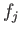are the Cartesian components of the forces acting on the various particles making up the system. Thus,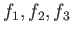are the components of the force acting on the first particle,the components of the force acting on the second particle, et cetera. Using Equation (B.2), we can also write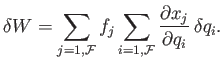(B.4)

The previous expression can be rearranged to give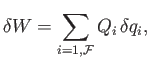(B.5)

where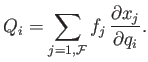(B.6)

Here, the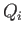are termed generalized forces. More explicitly,is termed the force conjugate to the coordinate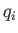. Note that a generalized force does not necessarily have the dimensions of force. However, the product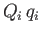must have the dimensions of work. Thus, if a particularis a Cartesian coordinate then the associatedis a force. Conversely, if a particularis an angle then the associatedis a torque.

Suppose that the dynamical system in question is energy conserving. It follows that(B.7)

for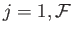, whereis the system's potential energy. Hence, according to Equation (B.6),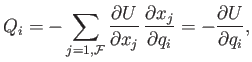(B.8)

for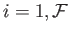.Next: Lagrange's Equation Up: Classical Mechanics Previous: Generalized Coordinates
Richard Fitzpatrick 2016-01-25